# A projectile is fired straight upward from the Earth's surface at thean initial speed equal to one third the escape speed. (a) Ignoring air resistance,determine how far from the center of the Earth the projectile travels beforeStopping momentarily . (b) What is the altitude of the projectile at this instant?uth Pole withP 7.47(a) One of the moons of Jupiter, named Io, has an orbital radius of 4.22 x 108 mand a period of 1.77 days. Assuming the orbit is circular, calculate the mass ofJupiter. (b) The largest moon of Jupiter, named Ganymede, has an orbital radius of1.07 x 10° m and a period of 7.16 days. Calculate the mass of Jupiter from thisdata. (c) Are your results to parts (a) and (b) consistent? Explain. an initial speed equal to one third the escape speed. (a) Ignoring air resistance,determine how far from the center of the Earth the projectile travels beforestopping momentarily . (b) What is the altitude of the projectile at this instant?P 7.47(a) One of the moons of Jupiter, named Io, has an orbital radius of 4.22 x 10% mand a period of 1.77 days. Assuming the orbit is circular, calculate the mass ofJupiter. (b) The largest moon of Jupiter, named Ganymede, has an orbital radius of1.07 x 10' m and a period of 7.16 days. Calculate the mass of Jupiter from thisdata. (c) Are your results to parts (a) and (b) consistent? Explain.

Question

Can you help me on p. 747. I am confused.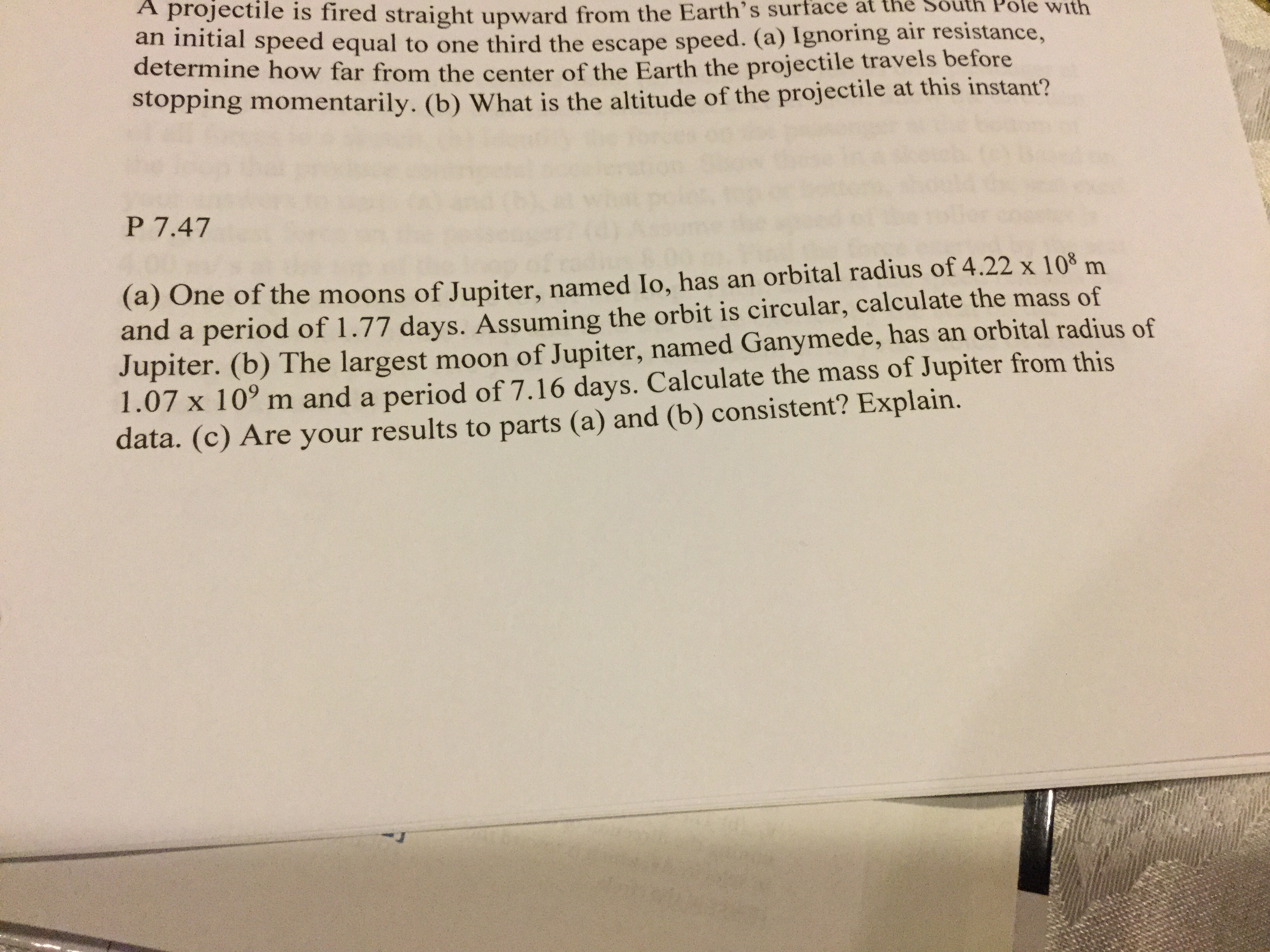help_outlineImage TranscriptioncloseA projectile is fired straight upward from the Earth's surface at the an initial speed equal to one third the escape speed. (a) Ignoring air resistance, determine how far from the center of the Earth the projectile travels before Stopping momentarily . (b) What is the altitude of the projectile at this instant? uth Pole with P 7.47 (a) One of the moons of Jupiter, named Io, has an orbital radius of 4.22 x 108 m and a period of 1.77 days. Assuming the orbit is circular, calculate the mass of Jupiter. (b) The largest moon of Jupiter, named Ganymede, has an orbital radius of 1.07 x 10° m and a period of 7.16 days. Calculate the mass of Jupiter from this data. (c) Are your results to parts (a) and (b) consistent? Explain. fullscreen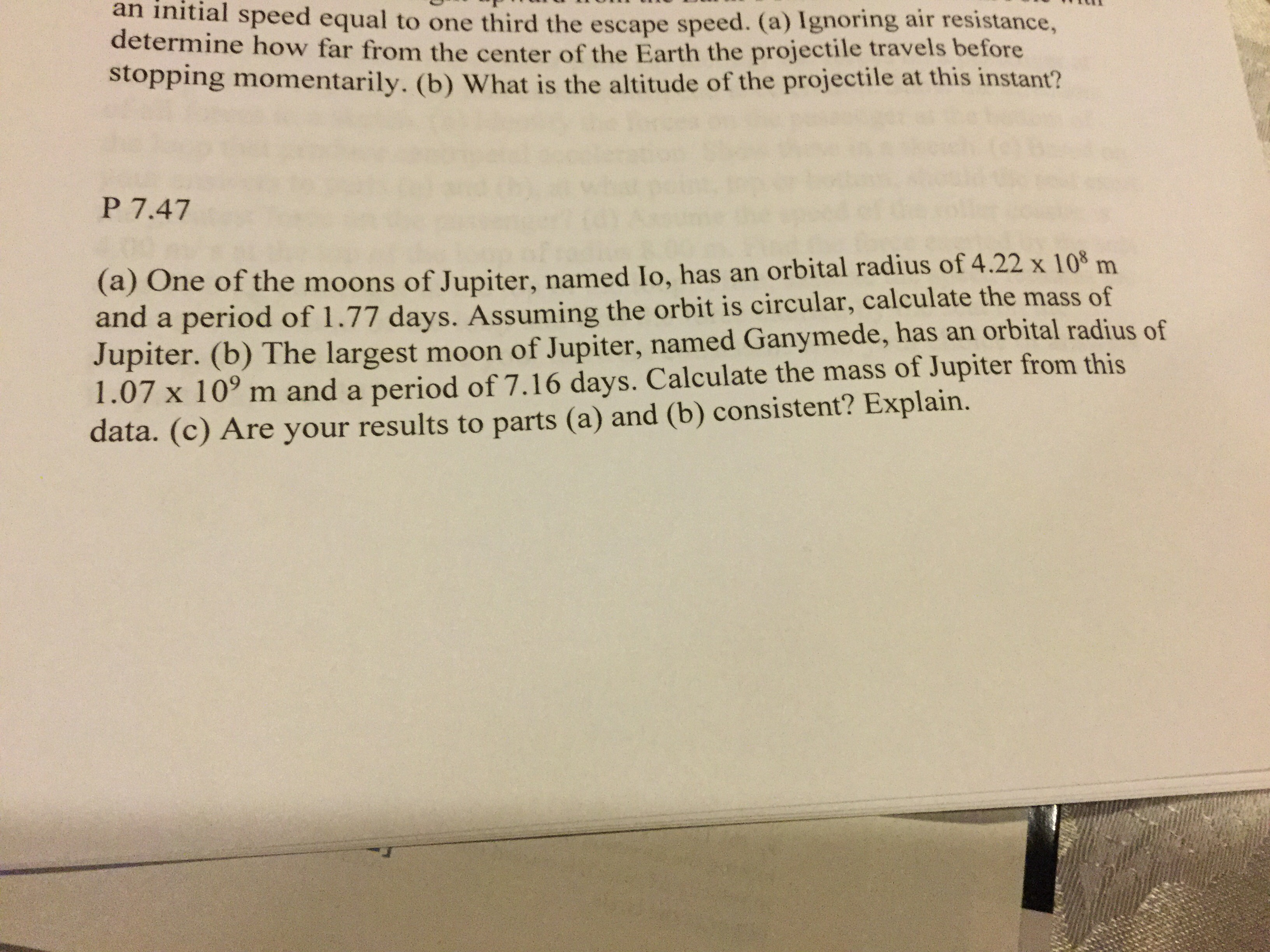help_outlineImage Transcriptionclosean initial speed equal to one third the escape speed. (a) Ignoring air resistance, determine how far from the center of the Earth the projectile travels before stopping momentarily . (b) What is the altitude of the projectile at this instant? P 7.47 (a) One of the moons of Jupiter, named Io, has an orbital radius of 4.22 x 10% m and a period of 1.77 days. Assuming the orbit is circular, calculate the mass of Jupiter. (b) The largest moon of Jupiter, named Ganymede, has an orbital radius of 1.07 x 10' m and a period of 7.16 days. Calculate the mass of Jupiter from this data. (c) Are your results to parts (a) and (b) consistent? Explain. fullscreen
check_circle

Step 1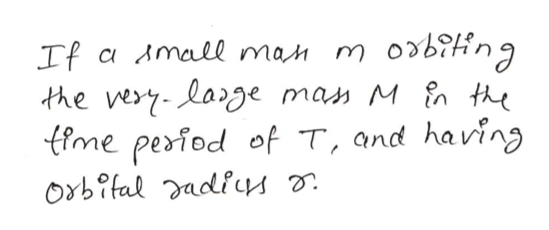help_outlineImage TranscriptioncloseIf a Amall man m obiin the very-lage mas M n the ffme period of T, and having Orbifal adiuu fullscreen
Step 2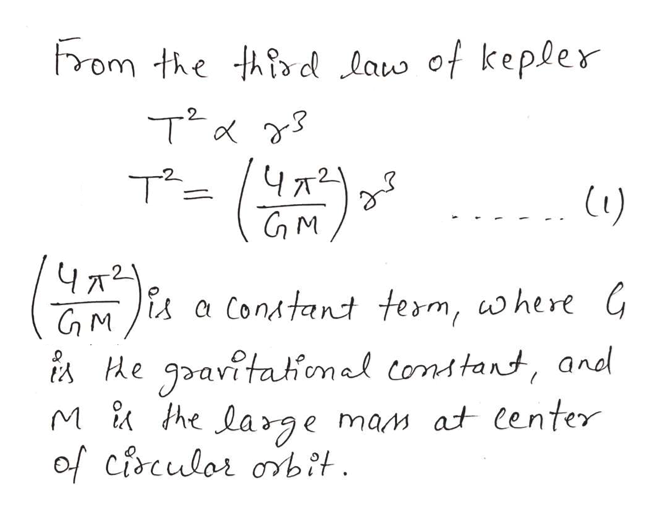help_outlineImage TranscriptioncloseFrom the thrd law of kepler Чла GM Чя a Conatant term, where G iA Me gavitahonel constant, and he large mam at lenter of cisculor obit fullscreen
Step 3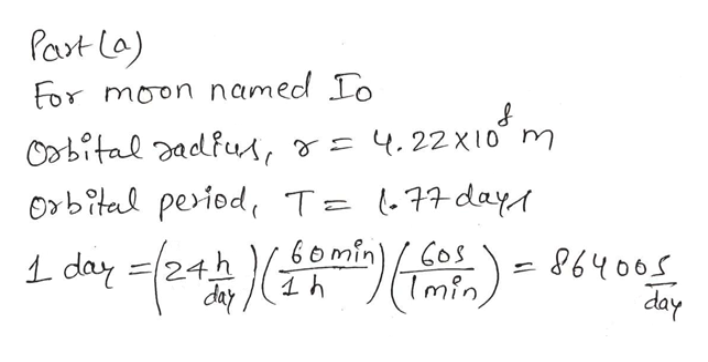help_outlineImage TranscriptionclosePart La) For moon named Io Obital adfus, &= 4.22X10 m Oxbital period T= 177day 6o min Cos ৪6५०० day L day 1 (Imin day fullscreen

### Want to see the full answer?

See Solution

#### Want to see this answer and more?

Solutions are written by subject experts who are available 24/7. Questions are typically answered within 1 hour.*

See Solution
*Response times may vary by subject and question.
Tagged in

### Gravitation